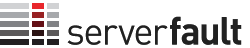0

## Please login or register to vote for this query.

(click on this box to dismiss)Q&A for system and network administrators

``````SELECT
COUNT(DISTINCT p.id) as posts,
COUNT(c.id)/COUNT(DISTINCT p.id) as ComPerPost,
(SELECT COUNT(v.id) FROM votes v where v.VoteTypeId = '2') as votesUp,
(SELECT COUNT(v.id) FROM votes v where v.VoteTypeId = '3') as votesDown,
COUNT(CASE when v.votetypeid in ('15') then v.id end) as votesDel,
CAST(
CAST(COUNT(CASE when v.VoteTypeId = '2' then v.id end) as DECIMAL(20,5))
/
CAST(COUNT(DISTINCT p.id) as DECIMAL(20,5))
as DECIMAL(20,5)) as voteweightUp,
CAST(
CAST(COUNT(CASE when v.VoteTypeId = '3' then v.id end) as DECIMAL(20,5))
/
CAST(COUNT(DISTINCT p.id) as DECIMAL(20,5))
as DECIMAL(20,5)) as voteweightDown
FROM posts p
left join votes v
on p.id = v.postid
left join comments c
on p.id = c.postid
WHERE p.creationdate > '2014-06-01'``````

### Enter Parameters

Options:
-Hold tight while we fetch your results
:records returned in :time ms:cached